An Improved Hyperbolic Summation Imaging Algorithm for Detection ofthe Subsurface Targets | OMICS International
Journal of Remote Sensing & GIS

# An Improved Hyperbolic Summation Imaging Algorithm for Detection ofthe Subsurface Targets

 Alireza Akbari1, Mirsattar Meshinchi-Asl1* and Mohmmad-Ali Riyahi2 1Department of Geophysics, Science and Research Branch, Islamic Azad University, Tehran, Iran 2Institute of Geophysics, University of Tehran, Tehran, Iran Corresponding Author : Mirsattar Meshinchi-Asl Department of Geophysics Science and Research Branch Islamic Azad University, Tehran, Iran Tel: +91 80 40387777 Fax: +91 80 40387600 E-mail: [email protected] Received August 28, 2014; Accepted October 20, 2014; Published October 24, 2014 Citation: Akbari A, Meshinchi-Asl M, Riyahi MA (2014) An Improved Hyperbolic Summation Imaging Algorithm for Detection of the Subsurface Targets. J Geophys Remote Sensing 3:132. doi:10.4172/2169-0049.1000132 Copyright: © 2014 Akbari A, et al. This is an open-access article distributed under the terms of the Creative Commons Attribution License, which permits unrestricted use, distribution, and reproduction in any medium, provided the original author and source are credited.

Visit for more related articles at Journal of Remote Sensing & GIS

#### Abstract

In Ground penetrating Radar (GPR) imaging a single point target appears as a hyperbolic curve in the space-time image. In this study, for focusing hyperbolic curves in GPR images, we present an improved hyperbolic summation (HS) focusing technique based on cross-correlation receiving GPR data. First, the formulation of the proposed algorithm is presented. Second, for improve quality images result of HS imaging algorithm a weight factor is designed by analyzing the statistical character of receiving data for each point in region imaging. Third, this proposed algorithm applied on numerically and experimental GPR data and results shown that the proposed hyperbolic summation imaging algorithm superiority concentrate hyperbolic curves in GPR images and images result have a good quality and resolution. In order to quantitatively describe the imaging result for the effect of artifact suppression, focusing parameter is evaluated.

 Keywords Ground penetrating radar (GPR) imaging; Hyperbolic Summation (HS) Introduction GPR is an important nondestructive remote sensing tool which used both military and civilian fields. Recently GPR imaging has drawn lots of attention in detection subsurface shallow small targets such as landmines and Unexploded Ordnance and imaging behind the wall for security applications [1-4]. Depending on the application, different scanning schemes, namely, A-scan, B-scan, and C-scan, are being employed. This static measured data collected at single point is called an A-scan. In the B-scan measurement situation, a downward looking GPR antenna is moved along a straight path on the top of the surface while the GPR sensor is collecting and recording the scattered field at different spatial positions as shown in Figure 1a. The data collection in a typical GPR operation can be either employed in the time domain by recording the scattered response of a time-domain pulse or in the frequency domain by recording the frequency response of the scattered field. For the former case, the twodimensional (2D) space-time GPR image (x,) is attained by conveying a time domain pulse towards the surface at consecutive, distinct synthetic aperture points. For the latter case, an Inverse Fourier Transform (IFT) operation should be accommodated to carry the collected data from the spatial-frequency domain to space-time GPR image. The common goal in a typical GPR image is to display the information of the spatial location and the reflectivity of an underground object. Therefore the main challenge of GPR imaging technique is to devise an image reconstruction algorithm that provides high resolution and good suppression of strong artifacts and noise. The depth of resolution achieved by the transmitted signal’s frequency diversity. The resolution along the direction recording data is attained by the synthetic processing of the received data collected at different spatial points of the B-scan. While a fine resolution in the depth axis is usually easy to get by utilizing a wideband transmitted signal, the resolution along the scanning direction is much harder to realize and requires special treatment. The GPR can either be bistatic with both a transmitted and receiver antenna or monostatic with a single transmitted and receiver antenna. For homogeneous mediums, the phase of the received signal is directly proportional to the trip distance that EM wave possesses. Therefore, the back-scattered signal from a point scatterer experience different roundtrip times and distances while the radar is moving along a straight line for the monostatic arrangement as depicted in Figure 1a. For each discrete point on this aperture, the backscattered signal can be collected within a frequency bandwidth (A-scan) such that one dimensional (1- D) range-profile. Then, one can easily construct a 2-D B-scan GPR image in the spatial-time domain by putting all range profiles side by side. After applying these procedures, a point scatterer shows up as a hyperbolic parabola in this 2-D B-scan GPR image, as shown in Figure 1b. The shape of this hyperbola depends on the depth of the buried object, the beam-width of the radar antenna, and the relative electric permittivity of the ground medium . The true location of the object is in fact at the top of this hyperbola. With this hyperbolic curve, the resolution along the synthetic aperture direction shows undesired low resolution features owing to the tails of hyperbola. However, highly accurate information about the size, electromagnetic (EM) reflectivity and depth of the buried objects is necessary in most GPR applications. Therefore hyperbolic curve behavior in the space-time GPR image often desired to be transformed to a focused pattern that shows the object's true location and size together with its EM scattering. The common name for this task is called migration or focusing and recently many image focusing algorithms have been developed. Synthetic aperture imaging methods used in GPR applications can be sorted into two main categories; namely the back-propagation and the back-projection methods. The first class is formulated through various algorithms including the Kirchhoff waveequation , the phase-shift method , the finite-difference method  and the frequency-wavenumber algorithm . Another class is formulated through the geometrical approach and includes the backprojection  and hyperbolic summation  algorithms. Hyperbolic Summation (HS) imaging algorithm is a simple but effective method to focus such hyperbolic curve undesired that successfully applied especially in seismic application. Ozdemir et al.  introduced hyperbolic summation technique for focusing undesired hyperbola curves in GPR images. However, the information included in one-directional (1-D) data located at the time-delay curve was not used sufficiently, and so the imaging quality is not as good. In this paper, an improved Hyperbolic Summation (HS) imaging algorithm based on cross-correlation between receiving signals for artifacts suppression is presented. Then to improve the quality of proposed HS imaging algorithm result a weight factor is designed for each point in region imaging. The proposed improved HS algorithm applied to numerically generated GPR data and real B-scan GPR images and resultant focused GPR images are presented. In order to quantitatively describe the imaging result for the effect of suppression artifacts, a focusing parameter evaluated. Standard Hyperbolic Summation Algorithm Method A typical GPR system collects EM reflectivity of the subsurface objects together with various cluttering effects caused mostly by airto- ground interface and in homogeneities within the ground medium . The 2-D imaging configuration of monostatic GPR is shown in Figure 2. We assume that the radar antenna is put very close to air– ground surface and the ground medium is homogeneous as well. This assumption assures that spatial-time GPR data can easily be converted to spatial-spatial GPR data by applying z=vt, where v is the velocity of the EM wave inside the ground medium, t is time, and z is the depthaxis. The region imaging is divided into two regions by z=0. The upper region is air characterized by permittivity ε1 = ε0 and permeability μ1=μ0 where ε0 and μ0 are parameters permittivity and permeability in air, respectively. The lower region is ground and characterized with homogeneous soil with relatively permittivity ε 2= ε and relatively permeability μ2=μ0 For the sake of simplicity, we assumed that the conductivity in air and homogeneous soil is zero. In this monostatic GPR system, the antenna located at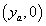and synthesizes an aperture on y axis, with M element. The whole scanning length of targets is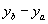. As the transmitter/receiver antenna pair move along the synthetic aperture line on z=0 with the interval Δy, backscattering signals at the each focal aperture point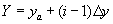, that i=1,2…,M, can be collected. The currently concerned antenna position in the monostatic GPR array is shown black triangle with the sequence number i, whose coordinate is (Yi,0), while other M-1 antenna position are represented by white triangles Under these assumptions, the backscattered electric field from a point scatterer buried at zp for an A-scan process in frequency domain can be simply written as (1)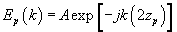(1) Where A is the complex EM scattering value of the buried scatterer and k is the wave-number inside the ground. It is obvious that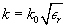,where k0 is the wave-number in free space and εr is the dielectric constant of the ground. The scattered field from subsurface environment is collected along a straight path via Step Frequency Radar (SFR) system that the frequency is varied within a certain bandwidth of fBW for N equally spaced steps either for monostatic configuration. Then, 1-D A-scan depth-profile can be constructed by taking the 1-D Inverse Fourier Transforming (IFT) of the 1-D frequency data as shown (2)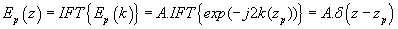(2) That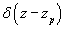is the impulse function. It is obvious that a peak of amplitude A, located at z=zp where the point scatterer actually lies. Similarly, traditional 2-D space-depth B-scan GPR image is obtained by taking the 1-D Inverse Fourier Transforming (IFT) of the 2-D spatial-frequency data. According to Fourier theory, depth resolution is given by (3)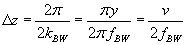(3) That kBW is the bandwidth in wave-number domain and v is the speed of light inside the ground medium. For homogeneous and lossless mediums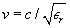, where c is the speed of light in vacuum. The depth extend; zmax is then can be calculated via (4)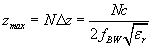(4) When the radar is moving on a synthetic aperture along a 1-D vector Y, a target point located at (yp,zp) shows up as a hyperbola whose depth equation can be written as (5)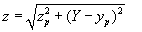(5) Noting that a B-scan GPR image is obtained by the summation of finite number of hyperbolas that correspond to different targets point on the buried object(s) that it is possible to resolve these points. For each pixel point (yi,zi) in the original 2-D B-scan GPR image, we find the hyperbolic template for the synthetic aperture vector Y using (6)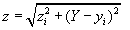(6) We trace the image data for the pixels under this hyperbolic template. However, for each pixel point, we have a 1-D data Ei (y) that i=1,2…,M is the same as the total number of sampling points in Y. Then, the value of pixels point in the final image I (x,z) can be formulated as (7)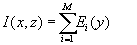(7) We then record the calculated values to the single pixel at (yi,zi) in the new 2-D GPR image. Therefore, a hyperbolic behavior in the original image is, in fact, mapped to a single image data point at (yi,zi) that contains the summation of the values point under this hyperbolic template in the new image. We successively repeat the same procedure until all the pixels in the original 2-D GPR image are covered. Improved Proposed HS Algorithm Modified cross-correlation HS One of the disadvantages of the HS imaging algorithm introduced that the level of the artifacts and noises energy in the imaging results is high. The existence of noises and artifacts decrease the contrast between objects and non-objects in imaging results. In fact more information can be obtained from the original receiving signal. The based on crosscorrelation to decreasing noises and suppression artifacts, proposed an improved HS algorithm for GPR application. At this kind of improved algorithm, value of the pixel point will not be summed directly as (7). Instead, similar CMI method [11,12], we will first calculate the crosscorrelation of values of between each focal point in M transmitter/ receiver position and then summation is made to take the imaging result of pixel point as follow(8):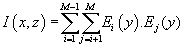(8) By this additional step, noises and artifacts in imaging result will be suppressed effectively. Design weight factor The value of standard deviation and mean 2D GPR that contain buried target reflected waves are greater than 2D GPR data that do not contain buried target reflected waves . So the ratio of the mean value to the standard deviation of focal point target is much bigger than corresponding value of the other focal point where there are no real targets. Thus with this characteristic can be modified the standard HS imaging algorithm. By charactering a weight factor ω (yn,zm) result of the imaging quality can be improved using mean (9) and standard deviation (10).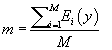(9)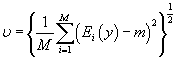(10) The weighting factor can be design by (11):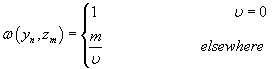(11) Now final improved proposed HS imaging algorithm with using cross-correlation between received data and weight factor is designed by analyzing the statistical character of receiving data, given by (12).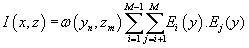(12) The Implementation of the Algorithm The steps of implementation the improved proposed HS imaging algorithm can be expressed as: 1. The scattered field from subsurface environment is collected along a straight path at M element. 2. For each pixel point (yi,zi) in the original B-scan GPR image, we find the hyperbolic template for the synthetic aperture vector. 3. Trace the image data for the pixels under this hyperbolic template. 4. For standard HS imaging algorithm, a summation is made to take the imaging result as shown (7) 5. For improved HS imaging algorithm, calculation crosscorrelation to utilize the relativities between M receiving signal as follow (8) 6. The weight factor ω (yn,zm) is calculated for each pixel point. 7. The final result in the improved HS imaging algorithm can be taking with (12). 8. Repeat the steps up to cover all of point in region imaging. Simulation and Experiment’s Results The validity and effectiveness of proposed HS algorithm are tested via simulated and real measured data. Simulation results In this section we describe the EM computer model that closely simulates the operation GPR for underground target detection. This model uses the Finite Difference Time Domain (FDTD) method for EM field calculation. A simulation consists of exciting one transmitter with current pulse and receiving the time domain y component of the electric field at the receiver location. This simulates a monostatic radar configuration and is repeated separately for each transmitter/receiver pair. The received signals are including the wave propagation directly between the transmitter and receiver, the EM wave reflected by airground interface and EM wave scattered by underground anomaly. Since we are interested only received signal from buried target, we should be cancelled the direct and interface reflected signals. Here with using monostatic arrangement, the direct wave is cancelled and for remove air- ground interface reflected signals have been used mean subtracted method . For the EM calculation of scattering from underground region a CST microwave imaging simulator is employed. This simulator can successfully estimate EM scattering for any medium and all targets that buried there with FDTD method. The backscattered EM signature is collected along synthetic aperture in y direction for a total 61 discrete spatial points. The separation between two antenna positions is 5 cm. The antennas are placed at 5 cm above air-ground interface. The entire synthetic aperture is 3.05 m along y dimension. The frequency is varied from 1 to 4 GHz with a 2.5 GHz central frequency. Three landmines with identical size were located at different locations as the buried targets. These landmines with 12 cm in diameter and 6 cm in length are put horizontally at (-0.7,-0.5), (-0.50,-0.2) and (-0.7,0.2) in meters. These landmines buried in depths 10 cm, 5 cm and 15 cm respectively. This targets buried in homogeneous soil with the dielectric constant εr =13 . This dielectric property is characteristic of wet sandy soil . The monostatic GPR arrangement geometry and three landmines positions show in Figure 3a and 3b. The B-scan path is mainly along the y-axis, through the center of the landmines region. Each scan position is denoted by black dot in Figure 3b. Each landmine displays its hyperbola shaped range profiles as radar approaches and then eventually passes each target. In addition to the main response from each landmine, there is some late time response, which is generated from wave travelling around the body of landmine. As plotted in Figure 4, the traditional GPR image obtained in 2D spatial-time backscattered data after removing air-ground interface effect with mean subtracted method. As expected, the image is defocused hyperbolically due to different round-trip distance targets to antennas while radar is moving along straight line for the monostatic arrangement. For focusing hyperbolas due to buried landmines the standard and the proposed improved algorithms applied and images results from standard HS algorithm and improved HS algorithm are shown at Figure 5. It is obvious that Figure 5a has much more noise and artifacts than Figure 5b. Therefore the proposed improved HS imagine algorithm can obtain a better imaging result with the good artifacts suppression. In order to quantitatively describe the effect of artifacts suppression, by standard and improved BP algorithms, we evaluated Integrated Side Lobe Ratio (ISLR) parameter. This parameter is the ratio of the energy in the side lobes to that contained in the main lobe that defined as (13):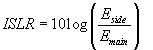(13) Where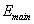and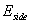are the energy of main lobe of object and the energy of side lobe of object that is defined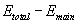and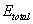is the energy of the image. The calculated ISLR parameter for Figure 5b and 5a are -6.4446 dB and -3.8979 dB respectively, the ISLR parameter decreases by 2.5467 dB. For better understand of decreasing and suppression of artifacts with standard and improved algorithms, two profiles at the peak point along y and depth axes of the imaging results are displayed in Figure 6. An average decline of suppression is 2.5 dB in both profiles are shown in Figure 6a and 6b. Experimental Results To test the standard and improved proposed HS imaging algorithm a GPR survey conducted. The goal of this survey is imaging two buried metallic bars (PEC) in concrete block. The equipment evaluated in this work is a RAMAC/GPR (MALA Geosciences) and a shielded ground- coupled antenna with a nominal central frequency of 2.3 GHz. In this experiment, two identical iron bars were buried at nearly 10 and 13 cm below the homogeneous concrete block. The two metallic bars are put along the x-axis. The relative permittivity concrete block is 4.5. Under monostatic configuration, the backscattered field data is collected along a synthetic aperture length 80 cm. Antenna was located just above the concrete surface and headed toward the buried object while it is moving along the aperture. Assuming that the bars routes are generally known a-priori, the B-scan measurements along the longitudinal direction of the bars were taken. The acquired time series B-scan data were then processed to locate the buried bars. Figure 7 shows the imaging result of GPR received data after preprocessing. As expected, the image result is defocused hyperbolically due to different round-trip distance targets to antennas while radar is moving along straight line for the monostatic arrangement. Figure 8 shows the imaging result of standard and improved proposed HS algorithm respectively. It is obviously that Figure 8a has much more artifact than in Figure 8b. It is obvious that image result from improved HS algorithm is more concentrated around the true location of the metallic bars and the reflection from other surfaces are well suppressed as they are not visible within the dynamic range of figure. Thus superiority in artifact suppression and resolution of improved proposed HS algorithm over the standard one is conspicuous. The calculated ISLR parameter for Figure 8b and 8a are -10.5527 dB and -8.3118 dB respectively, the ISLR parameter decreases by nearly 2.2409 dB. For better understand of decreasing and suppression of artifacts with standard and improved algorithms, two profiles at the peak point along y and depth axes of the imaging results are displayed in Figure 9. An average decline of suppression is 2.25 dB in both profiles are seen in Figure 9a and 9b. Conclusion The paper presented an improved of the cross-correlation HS algorithm for GPR imaging. This improved proposed HS imaging algorithm has significantly suppressed noises and artifacts in the imaging result of the standard HS algorithm. By using a weight factor that designed by statistical character received data, imaging result shows a better performance for focusing quality. Simulation results and the real data imaging demonstrated it validity in GPR high resolution imaging. In order to quantitatively describe the imaging result for the effect of artifact suppression with proposed improved HS algorithm, focusing parameter is evaluated. Future work should focus on improving the proposed algorithm to adapt to disperse medium and multi-layer scenario. References Daniels DJ (2004) Ground penetrating radar 2nd ed. IEE Radar, Sonar and Navigation Series 15. The Institution of Electrical Engineers: London, UK, 1-19. Peters L, Daniels DJ, Young JD (1994) Ground penetrating radar as a subsurface environmentalsensing tool. Proc IEEE 82: 1802-1822. Vitebskiy S, Carin L, Ressler MA, Le FH (1997) Ultra-Wideband, Short pulse ground penetrating radar: simulation and measurement. IEEE Trans Geosci Remote Sens 35: 762-772. Carin L, Geng N, Sichina J, Nguyen, L (1999) Ultra-wide band synthetic aperture radar for mine-field detection. IEEE Trans Antennas Propag 41: 18-33 . Schneider WA (1978) Integral formulation for migration in two and three dimensions. Geophysics 43: 49-76 . Gazdag J (1978) Wave equation migration with the phase-shift method. Geophysics 43: 1342-51 . Claerbout JF (1972) Downward continuation of moveout-corrected seismograms. Geophysics 37: 741-768 . Ozdemir C, Demirci S, Yigit E (2008) Practical algorithms to focus B-scan GPR images: theory and application to real data. Progress in Electromagnetics Research B 6: 109-122 . Miller C, Oristaglio M, Beylkin GA (1978) A newslant onseismic imaging:Migration integral geometry. Geophysics 52: 943-64 . Ozdemir C, Lim S, Ling H (2004) A synthetic aperture algorithm for ground-penetrating radar imaging. Microwave Opt Tech Lett 42: 412-414 . Fear EC, Li X, Hagness SC, Stuchly MA (2002) Confocal microwave imaging for breast cancer detection: Localization of tumors in three dimensions. IEEE Trans. Biomed Eng 49: 812-822 . Lim HB, Nhung TT, Li EP, Thang ND (2008) Confocal microwave imaging for breast cancer detection: Delay-multiply-and-sum image reconstruction algorithm. IEEE Trans. Biomed Eng 55: 1697-704 . Gu K, Wang G, Li J (2004) Migration based SAR imaging for ground penetrating radar systems. Proceedings of Radar 151: 317-325 . Mast JE, Johansson EM (1994) Three-dimensional ground penetrating radar imaging usingsynthetic aperture time-domain focusing. Advanced Microwave and Millimeter Wave Detectors 2275: 205-214 . Wiggins R (1985) Entropy guided deconvolution. Geophysics 50: 2720-2726 .

#### Figures at a glance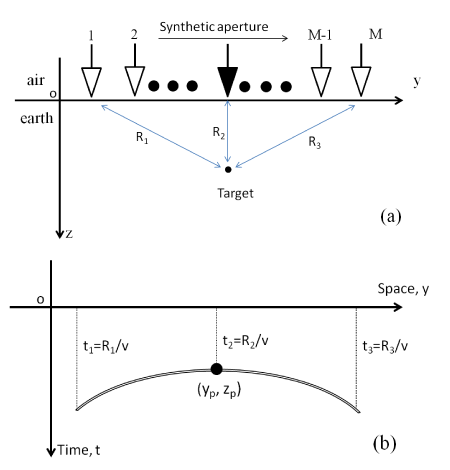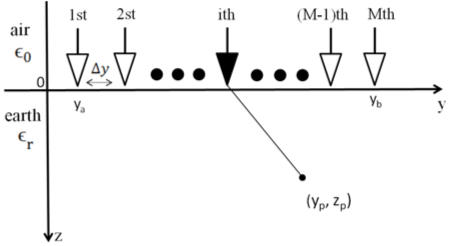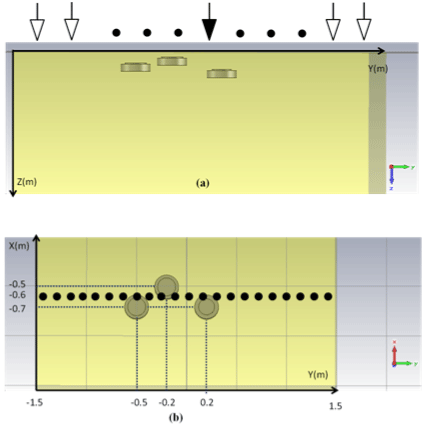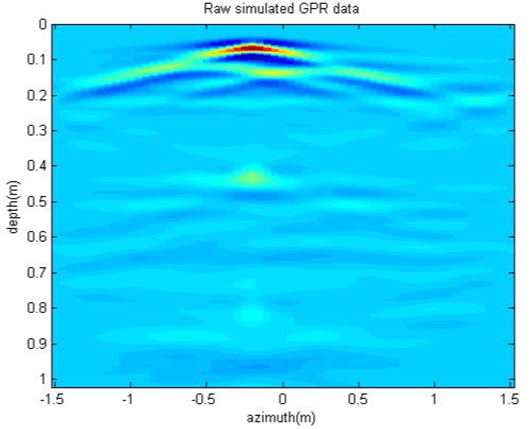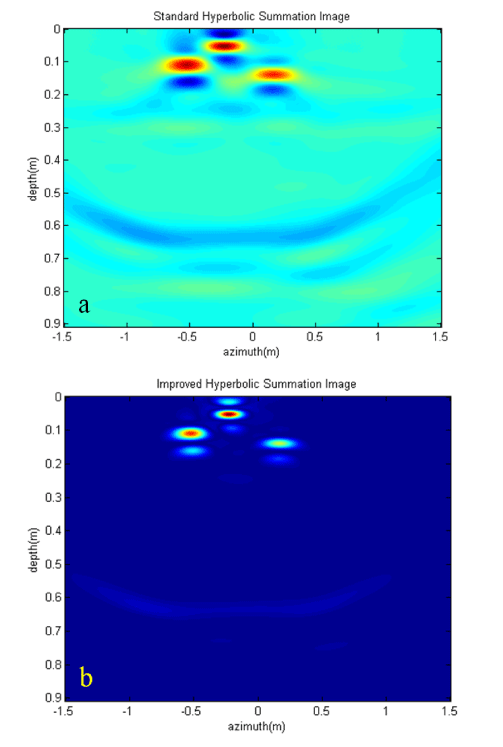Figure 1 Figure 2 Figure 3 Figure 4 Figure 5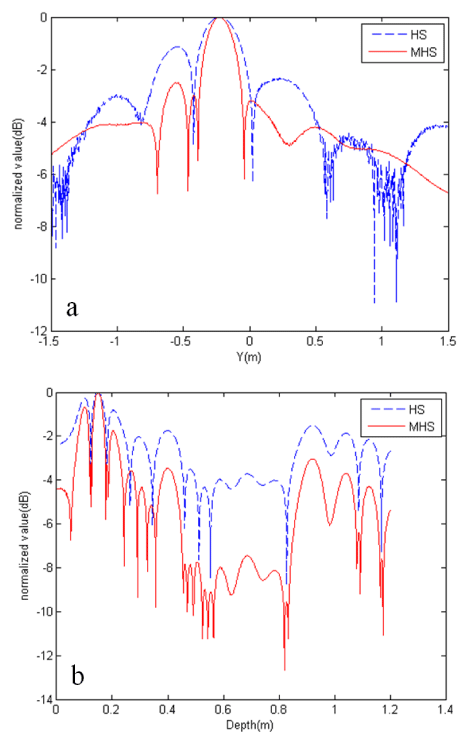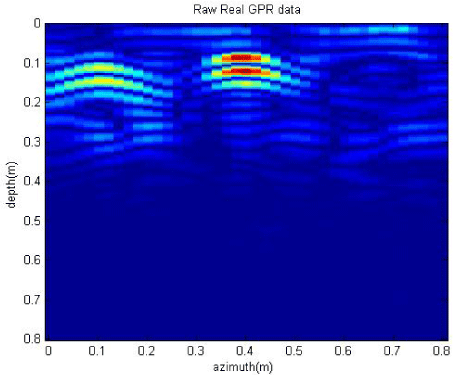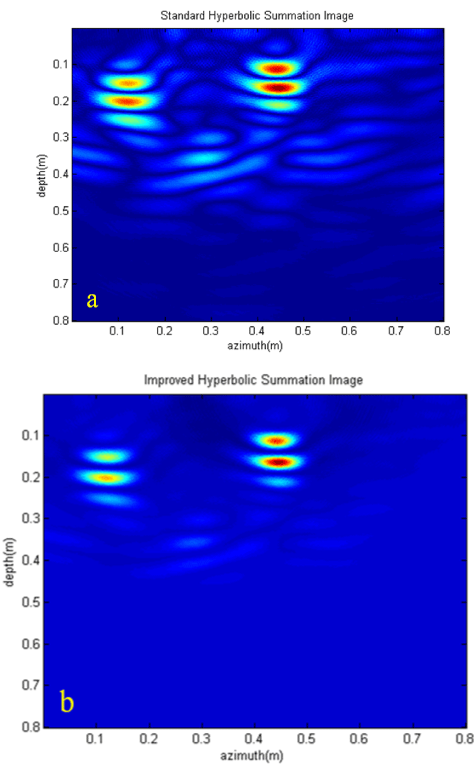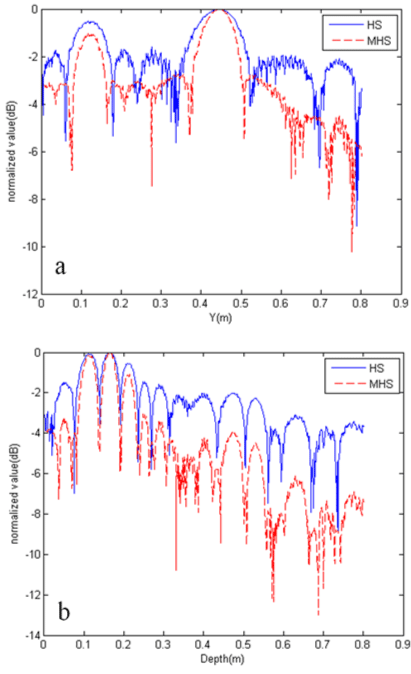Figure 6 Figure 7 Figure 8 Figure 9
Select your language of interest to view the total content in your interested language

### Article Usage

• Total views: 12636
• [From(publication date):
December-2014 - Jan 19, 2020]
• Breakdown by view type
• HTML page views : 8755Can't read the image? click here to refresh